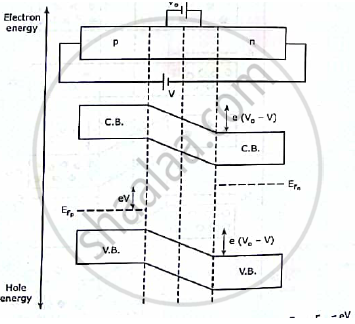# Draw the Energy Band Diagram of P-n Junction Diode in Forward and Reverse Bias Condition . - Applied Physics 1

Short Note

Draw the energy band diagram  of p-n junction diode in forward and reverse bias condition .

#### Solution

Forward biasing increases the electron density in the conduction band of the n-side .As a result the fermi level moves upwards .Similarly due to the increase in the hole density in the valence band of the p side ,the fermi level moves downwards. The fermi levels and are displaced relatively by an amount eV equal to the potential energy due to the applied voltage,V which cause the displacement.
The height of the conduction hill reduces by the same amount eV and becomes e(Vo-V).  Similarly  the height of the valence hill becomes -e(Vo- V). This makes the charge flow through the junction easier .Concept: Fermi Level Diagram for P-N Junction
Is there an error in this question or solution?